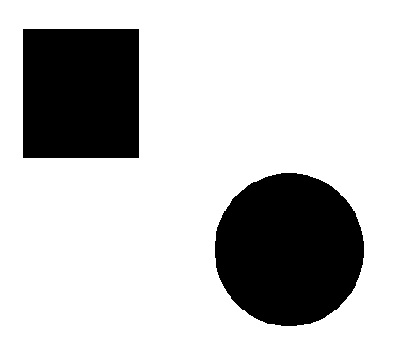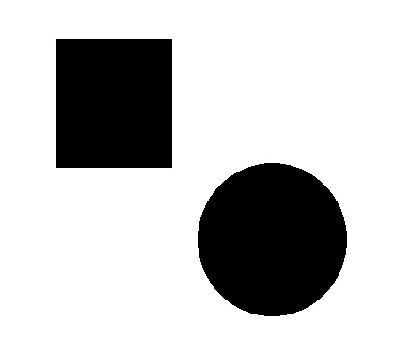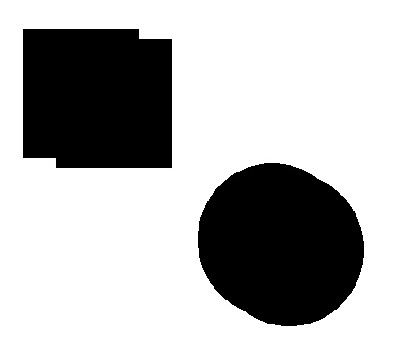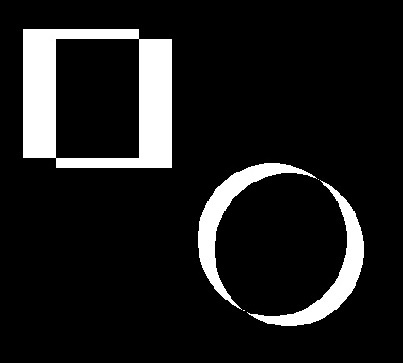# Bitwise Operations

We can perform various bitwise operations on images using OpenCV. Bitwise operations are very useful in image masking. In this tutorial, we will learn about how to perform bitwise operations like - AND, OR, XOR, and NOT on images.

### What is Bitwise Operator?

Bitwise operators are a set of operators in computer programming that perform operations on binary digits or bits. They are commonly used to perform manipulation of individual bits.

When we do image masking we take black(0) and white(255) as binary digits and perform operations accordingly.

### OR Operation

In OpenCV, the cv2.bitwise_or() function is used to perform OR operation of two images. OR operation works similarly to OR operation in mathematics. The operation is performed on the binary representation of the pixel values. Let’s understand this with some practical work.

Example:

Input 1Input 2Code:

``````import cv2
image = cv2.bitwise_or(image1, image2)
cv2.imshow('image', image)
cv2.waitKey(0)
cv2.destroyAllWindows()``````

Here, first we imported cv2 and then we used cv2.imread to read both images of the given path. Then we used  cv2.bitwise_or function to perform OR operation on the pixels of both images. At last, we used the cv2.imshow function to display the final result.

Result:

### And Operation

In OpenCV, the cv2.bitwise_and() function is used to perform AND operation of two images. It is also similar to AND operation of mathematics. In simple words, this operation is used to display the addition of areas from both images as output.

Example:

Input 1Input 2Code:

``````import cv2
image = cv2.bitwise_and(image1, image2)
cv2.imshow('image', image)
cv2.waitKey(0)
cv2.destroyAllWindows()``````

Here, first we imported cv2 and then we used cv2.imread to read both images of the given path. Then we used  cv2.bitwise_and function to perform AND operation on the pixels of both images.

### Result:XOR Operation

In OpenCV, the cv2.bitwise_xor() function is used to perform XOR operation of two images.

Example:

Input 1Input 2Code:

``````import cv2
image = cv2.bitwise_xor(image1, image2)
cv2.imshow('image', image)
cv2.waitKey(0)
cv2.destroyAllWindows()``````

Here, we used  cv2.bitwise_xor function to perform XOR operation on the pixels of both images.

Result:### NOT Operation

In OpenCV, the cv2.bitwise_not() function is used to perform the NOT operation on the image. it takes one image as input. NOT is used for the inversion of inputs.

Example:

Input 1​​​​​​​Code:

``````import cv2